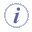# Part MeasuressmartVolume and smartWetarea refer to intermediate states of a solid. smartVolume does not compute the volume of each pad contained in a PartBody but the total volume.  Example: Given a PartBody containing 3 pads: The volume of Pad.1 = 0.1m3, The volume of Pad.2=0.1m3 and the volume of Pad.3=0.1m3. The Volume of Pad.3 displayed will be Pad.3=0.3M3. The volume of Pad.3=the Volume of Pad.1+ the volume of Pad.2+ the volume of Pad.3. Note that this applies also to smartWetarea (the total wet area is computed).
 smartVolume(elem: Solid, ...): Volume Returns the volume of a solid.Example Total_Volume= smartVolume(PartBody) smartWetarea(elem: Solid, ...) : Area Returns the wet area of a solid.Example Total_Area= smartWetarea(PartBody\Pad.1 )